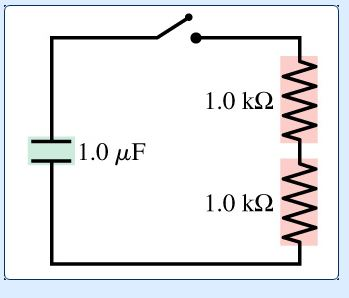# Problem: What is the time constant for the discharge of the capacitor in the figure?

###### FREE Expert Solution

Time constant:

$\overline{){\mathbf{T}}{\mathbf{=}}{\mathbf{R}}{\mathbf{C}}}$

Equivalent resistance for series resistors:

$\overline{){{\mathbit{R}}}_{\mathbf{e}\mathbf{q}}{\mathbf{=}}{{\mathbit{R}}}_{{\mathbf{1}}}{\mathbf{+}}{{\mathbit{R}}}_{{\mathbf{2}}}}$

R1 = R2 = 1.0 kΩ (1000Ω/1kΩ) = 1000Ω

Req = R1 + R2 = 1000 + 1000 = 2000 Ω

86% (210 ratings)###### Problem Details

What is the time constant for the discharge of the capacitor in the figure?